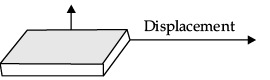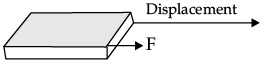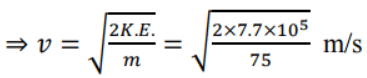Test: Work and Energy- Case Based Type Questions- 1

# Test: Work and Energy- Case Based Type Questions- 1 - Class 9

Test Description

## 10 Questions MCQ Test Physics for Class 9 - Test: Work and Energy- Case Based Type Questions- 1

Test: Work and Energy- Case Based Type Questions- 1 for Class 9 2023 is part of Physics for Class 9 preparation. The Test: Work and Energy- Case Based Type Questions- 1 questions and answers have been prepared according to the Class 9 exam syllabus.The Test: Work and Energy- Case Based Type Questions- 1 MCQs are made for Class 9 2023 Exam. Find important definitions, questions, notes, meanings, examples, exercises, MCQs and online tests for Test: Work and Energy- Case Based Type Questions- 1 below.
Solutions of Test: Work and Energy- Case Based Type Questions- 1 questions in English are available as part of our Physics for Class 9 for Class 9 & Test: Work and Energy- Case Based Type Questions- 1 solutions in Hindi for Physics for Class 9 course. Download more important topics, notes, lectures and mock test series for Class 9 Exam by signing up for free. Attempt Test: Work and Energy- Case Based Type Questions- 1 | 10 questions in 20 minutes | Mock test for Class 9 preparation | Free important questions MCQ to study Physics for Class 9 for Class 9 Exam | Download free PDF with solutions
 1 Crore+ students have signed up on EduRev. Have you?
Test: Work and Energy- Case Based Type Questions- 1 - Question 1

### Direction: Study the given figure and answer the following question.(i)(ii)Q. What will be the work done in the above situations? State whether it will be positive, negative or zero.

Detailed Solution for Test: Work and Energy- Case Based Type Questions- 1 - Question 1
POSITIVE WORK: The work done on an object is said to be positive work when force and displacement are in same direction.

Example: When an object moves on horizontal surface, force and displacement acts in same direction. So, work done is positive.

NEGATIVE WORK: The work done is said to be negative work when force and displacement are in opposite direction.

Example: When an object is thrown upwards,the force of gravity is in downward direction whereas displacement acts in upward direction.

ZERO WORK: The work done is said to be zero when force and displacement are perpendicular to each other or when either force or displacement is zero.

Example: When we hold an object and walk, the force acts in downward direction whereas displacement acts in forward direction.

Test: Work and Energy- Case Based Type Questions- 1 - Question 2

### Direction: Study the given figure and answer the following question. (i)(ii)Q. Write an expression for work in terms of force and displacement.

Detailed Solution for Test: Work and Energy- Case Based Type Questions- 1 - Question 2

when a force F displaces a body through a distance S in the direction of applied force, then the work done W on the body is given by the expression

Workdone = Force × displacement or W = F × S or W = Fs

Test: Work and Energy- Case Based Type Questions- 1 - Question 3

### Direction: Study the given figure and answer the following question.(i)(ii)Q. In case of negative work, the angle between the force and displacement is

Detailed Solution for Test: Work and Energy- Case Based Type Questions- 1 - Question 3
Work done can be positive,negative and zero. When a body is being lifted in the upward direction, the force of gravity is acting in the downward direction. But, the displacement of the body is in the upward direction. Since the angle between the force and displacement is 180 degrees, the work done by the gravitational force on the body is negative. Diagrammatic representation is given.Hence for negative work done, angle between force and displacement is 180°.
Test: Work and Energy- Case Based Type Questions- 1 - Question 4

Direction: Study the given figure and answer the following question.

(i)(ii)Q. Give reason for the same in each case.

Detailed Solution for Test: Work and Energy- Case Based Type Questions- 1 - Question 4
In figure, the direction of force, (F) and displacement are perpendicular to each other. There is no displacement in the direction of force so the work done is zero. In fig (ii), the direction of force F and displacement are in the same direction. Hence, work done by the force is positive.
Test: Work and Energy- Case Based Type Questions- 1 - Question 5

Direction: Study the given figure and answer the following question.

(i)(ii)Q. Define one joule of work.

Detailed Solution for Test: Work and Energy- Case Based Type Questions- 1 - Question 5
Joule, unit of work or energy in the International System of Units (SI); it is equal to the work done by a force of one newton acting through one metre. In electrical terms, the joule equals one watt-second—i.e., the energy released in one second by a current of one ampere through a resistance of one ohm.
Test: Work and Energy- Case Based Type Questions- 1 - Question 6

Direction: 75 kg missile is dropped downwards from an air plane, and has a speed of 60 m/s at an altitude of 850 m above the ground. Determine:

(a) The K.E. possessed by the missile at 850 m.

(b) The P.E. possessed by the missile at 850 m.

(c) The total mechanical energy possessed by the missile.

(d) The K.E. and velocity with which it strikes the ground.

Q. The K.E. possessed by the missile at 850 m.

Detailed Solution for Test: Work and Energy- Case Based Type Questions- 1 - Question 6
Given

Mass of the missile, m = 75 kg and Speed, v = 60 m/s

Therefore, K.E. = 1/ 2 mv2 = 1 /2 × 75 × 60 × 60 = 13500 J.

Test: Work and Energy- Case Based Type Questions- 1 - Question 7

Direction: 75 kg missile is dropped downwards from an air plane, and has a speed of 60 m/s at an altitude of 850 m above the ground. Determine:

(a) The K.E. possessed by the missile at 850 m.

(b) The P.E. possessed by the missile at 850 m.

(c) The total mechanical energy possessed by the missile.

(d) The K.E. and velocity with which it strikes the ground.

Q. The total mechanical energy possessed by the missile.

Detailed Solution for Test: Work and Energy- Case Based Type Questions- 1 - Question 7
Total mechanical energy of the missile = K.E. + P.E.

= 135000 + 637500 = 7.7 × 105J = 772500 J.

Test: Work and Energy- Case Based Type Questions- 1 - Question 8

Direction: 75 kg missile is dropped downwards from an air plane, and has a speed of 60 m/s at an altitude of 850 m above the ground. Determine:

(a) The K.E. possessed by the missile at 850 m.

(b) The P.E. possessed by the missile at 850 m.

(c) The total mechanical energy possessed by the missile.

(d) The K.E. and velocity with which it strikes the ground.

Q. The P.E. possessed by the missile at 850 m.

Detailed Solution for Test: Work and Energy- Case Based Type Questions- 1 - Question 8
Given,

Mass of the missile, m = 75 kg

Acceleration due to gravity, g = 10 m/s2 and Height, h = 850 m

Therefore, P.E. = m × g × h = 75 × 10 × 850 = 637500 J.

Test: Work and Energy- Case Based Type Questions- 1 - Question 9

Direction: 75 kg missile is dropped downwards from an air plane, and has a speed of 60 m/s at an altitude of 850 m above the ground. Determine:

(a) The K.E. possessed by the missile at 850 m.

(b) The P.E. possessed by the missile at 850 m.

(c) The total mechanical energy possessed by the missile.

(d) The K.E. and velocity with which it strikes the ground.

Q. The energy possessed by an object due to motion is ………

Detailed Solution for Test: Work and Energy- Case Based Type Questions- 1 - Question 9
Kinetic energy is the energy possessed by an object due to its motion. If an object is moving, then it has kinetic energy. If an object has kinetic energy, then it is moving.
Test: Work and Energy- Case Based Type Questions- 1 - Question 10

Direction: 75 kg missile is dropped downwards from an air plane, and has a speed of 60 m/s at an altitude of 850 m above the ground. Determine:

(a) The K.E. possessed by the missile at 850 m.

(b) The P.E. possessed by the missile at 850 m.

(c) The total mechanical energy possessed by the missile.

(d) The K.E. and velocity with which it strikes the ground.

Q. The K.E. and velocity with which it strikes the ground.

Detailed Solution for Test: Work and Energy- Case Based Type Questions- 1 - Question 10
K.E. at the ground = 7.7 × 105J K.E. = 1 /2 mv2,

Therefore, v2 = 2 K.E. / mVelocity with which missile strikes the ground = 143.2 m/s.

## Physics for Class 9

16 videos|69 docs|56 tests
Information about Test: Work and Energy- Case Based Type Questions- 1 Page
In this test you can find the Exam questions for Test: Work and Energy- Case Based Type Questions- 1 solved & explained in the simplest way possible. Besides giving Questions and answers for Test: Work and Energy- Case Based Type Questions- 1, EduRev gives you an ample number of Online tests for practice

## Physics for Class 9

16 videos|69 docs|56 tests